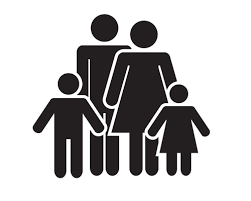# Grandfather 17981

Today, my grandfather is four times older than his grandson. My grandfather was six years younger than five times his grandson's age two years ago. How old is your grandson today?

v =  14

### Step-by-step explanation:

d =4v
d-2 = -6 +5(v-2)

d =4·v
d-2 = -6 +5·(v-2)

d-4v = 0
d-5v = -14

Row 2 - Row 1 → Row 2
d-4v = 0
-v = -14

v = -14/-1 = 14
d = 0+4v = 0+4 · 14 = 56

d = 56
v = 14

Our linear equations calculator calculates it.Did you find an error or inaccuracy? Feel free to write us. Thank you!

Tips for related online calculators
Do you have a system of equations and looking for calculator system of linear equations?
Do you want to convert time units like minutes to seconds?